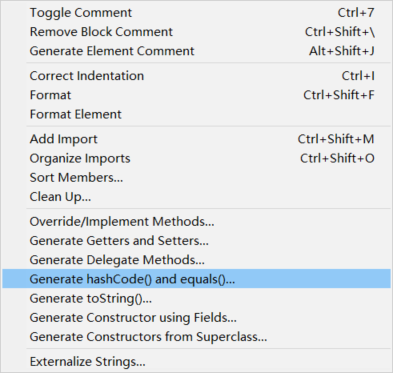Java基础知识点总结--从细节再看Java（八）--Set集合以及自定义类型未去重的解决

Set是Collection接口的子接口，模仿数学中的集合并对其进行抽象。我们先了解一下Set集合有什么样的特点：

• 最多包含一个null元素
• 没有索引
• 无序（存和读的顺序可能不同）

一、对Set集合的使用

Set与List一样，知道List怎么创建对象之后，我们也可以轻松了解到Set是如何创建对象的，来看代码：

	private static void method() {
// 创建集合对象
// HashSet<String> hs = new HashSet<String>();
Set<String> set = new HashSet<String>();

// 添加元素

// 遍历集合
Object[] objs = set.toArray();
for (int i = 0; i < objs.length; i++)
System.out.println(objs[i]);

for (String s : set)
System.out.println(s);

Iterator<String> it = set.iterator();
while (it.hasNext())
System.out.println(it.next());
}


HashSet是Set接口的一个实现类，可以用它来创建Set对象，同时Set的遍历与List相同，此处列举了遍历Set的三种方法。

二、Set存储自定义类型去重

class Student{
String name;
int age;
public Student(String name, int age) {
super();
this.name = name;
this.age = age;
}
@Override
public String toString() {
return "Student [name=" + name + ", age=" + age + "]";
}
}

public class HashSetDemo {

public static void main(String[] args) {

HashSet<Student> hs = new HashSet<Student>();
Student s1 =new Student("asv",12);
Student s2 = new Student ("ASD",15);
Student s3 = new Student ("ASD",15);

for(Student s:hs)
System.out.println(s);
}

}

    public boolean add(E e) {
return map.put(e, PRESENT)==null;
}

 //K key：要添加的新元素
public V put(K key, V value) {
//根据新添加元素的hashCode()返回值计算出hash值
int hash = hash(key);
int i = indexFor(hash, table.length);

//获取当前集合中的每一个元素
for (Entry<K,V> e = table[i]; e != null; e = e.next) {
Object k;

if (e.hash == hash && ((k = e.key) == key || key.equals(k))) {
V oldValue = e.value;
e.value = value;
e.recordAccess(this);
return oldValue;
}
}

modCount++;
//直接添加元素
return null;
}

put方法中有一个for循环，for循环中有一个判断语句，如果判断条件为真不添加新元素，否则添加新元素。因此，我们的重点也就是在这个if判断语句上，我们来看它的判断条件：e.hash == hash && ((k = e.key) == key || key.equals(k))。首先比较hash值，并且使用短路&，当hash值不一样时if语句结束添加新元素；如果hash值一样，再用==比较地址在或用equals方法进行比较，比较结果如果为true，则重复不在添加，否则添加新元素。

public class Student {
String name;
int age;
public Student() {
super();
}
public Student(String name, int age) {
super();
this.name = name;
this.age = age;
}
@Override
public String toString() {
return "Person [name=" + name + ", age=" + age + "]";
}

@Override
public int hashCode() {
return name.hashCode() + age ;
}
@Override
public boolean equals(Object obj) {
//对象一样，直接返回true ，提高效率
if (this == obj)
return true;
if (obj == null)
return false;

//使用getClass()比较，可以不用向下转型了   ，提高健壮性
if (getClass() != obj.getClass())
return false;

Student other = (Student) obj;
//比较年龄，不相等返回false
if (age != other.age)
return false;

//比较name，不相等返回false
if (!name.equals(other.name))
return false;

//都相等，返回true
return true;
}

}

三、自动生成hashCode方法和equals方法@Override
public int hashCode() {
final int prime = 31;
int result = 1;
result = prime * result + age;
result = prime * result + ((name == null) ? 0 : name.hashCode());
return result;
}
@Override
public boolean equals(Object obj) {
if (this == obj)
return true;
if (obj == null)
return false;
if (getClass() != obj.getClass())
return false;
Person other = (Person) obj;
if (age != other.age)
return false;
if (name == null) {
if (other.name != null)
return false;
} else if (!name.equals(other.name))
return false;
return true;
}

1. 使用质数计算hash值，质数与其他数相乘后，重复的概率小，结果唯一的概率更大。

2. 使用质数越大，冲突虽然减小，但计算速度变慢，31是哈希冲突和性能的折中。

3. JVM会自动对31进行优化，即31*i = (i<<5)-1.